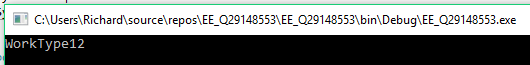# VB.net Extract value from datatable

Hi

I have a datatable that I need to extract a value from.
If I used SQL the query would be "Select WorkType From Employee Hours Where LineID = 12"

What VB.net code would I use to extract this value from a datatable called "dt".

Thanks
###### Who is Participating?
I wear a lot of hats...

"The solutions and answers provided on Experts Exchange have been extremely helpful to me over the last few years. I wear a lot of hats - Developer, Database Administrator, Help Desk, etc., so I know a lot of things but not a lot about one thing. Experts Exchange gives me answers from people who do know a lot about one thing, in a easy to use platform." -Todd S.

DeveloperCommented:
Using LINQ, you could do something like this:
``````Dim result = dt.Rows.Cast(Of DataRow).FirstOrDefault(Function(r) Equals(r("LineID"), 12))("WorkType")
``````
Or
``````Dim result = (From row In dt.Rows.Cast(Of DataRow) Where Equals(row("LineID"), 12) Select row("WorkType")).FirstOrDefault()
``````
Proof of concept -
``````Imports System.ComponentModel
Imports System.Runtime.CompilerServices

Module Module1
Sub Main()
Dim dt = Enumerable.Range(1, 20).Select(Function(i) New With {.ID = i, .WorkType = \$"WorkType{i}", .LineID = i}).ConvertToDataTable()
Dim result = dt.Rows.Cast(Of DataRow).FirstOrDefault(Function(r) Equals(r("LineID"), 12))("WorkType")
Console.WriteLine(result)
End Sub
End Module

Module Extensions
<Extension>
Public Function ConvertToDataTable(Of T)(ByVal source As IEnumerable(Of T)) As DataTable
Dim properties As PropertyDescriptorCollection = TypeDescriptor.GetProperties(GetType(T))
Dim table As DataTable = New DataTable()

For i As Integer = 0 To properties.Count - 1
Dim [property] As PropertyDescriptor = properties(i)
If [property].PropertyType.IsGenericType AndAlso [property].PropertyType.GetGenericTypeDefinition().Equals(GetType(Nullable)) Then
Else
End If
Next

Dim values(properties.Count - 1) As Object
For Each item As T In source
For i As Integer = 0 To properties.Count - 1
values(i) = properties(i).GetValue(item)
Next
Next

Return table
End Function
End Module
``````
Produces the following output --saige-

Experts Exchange Solution brought to you by